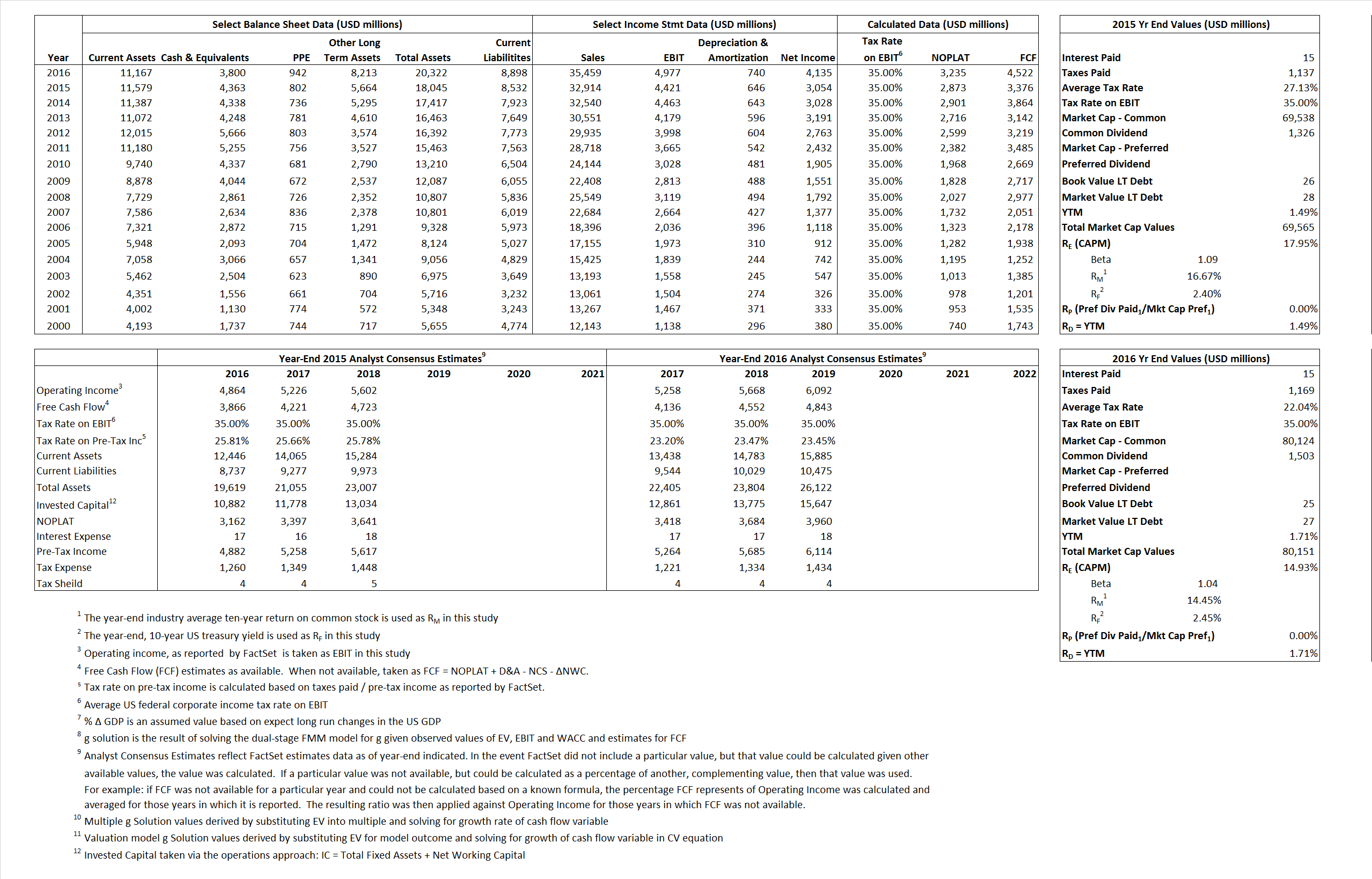# Accenture

## Analyst Listing

The following analysts provide coverage for the subject firm as of May 2016:

 Broker Analyst Analyst Email Raymond James Brian A. Gesuale brian.gesuale@raymondjames.com Cowen & Company Bryan C. Bergin bryan.bergin@cowen.com Deutsche Bank Research Bryan Keane bryan.keane@db.com Credit Suisse Charles Brennan charles.brennan@credit-suisse.com RBC Capital Markets Daniel Perlin daniel.perlin@rbccm.com Stifel Nicolaus David Grossman dgrossman@stifel.com Evercore ISI David Togut david.togut@evercoreisi.com Wells Fargo Securities Edward S. Caso edward.caso@wellsfargo.com SunTrust Robinson Humphrey Frank Atkins frank.atkins@suntrust.com Susquehanna Financial Group James Friedman james.friedman@sig.com Jefferies Jason Kupferberg jkupferberg@jefferies.com Cantor Fitzgerald Joseph D. Foresi jforesi@cantor.com BMO Capital Markets Keith Bachman keith.bachman@bmo.com Bernstein Research Lisa D. Ellis lisa.ellis@bernstein.com CRT Capital Group Moshe Katri mkatri@sterneageecrt.com

## Primary Input Data## Derived Input Data

### Equational Form

Net Operating Profit Less Adjusted Taxes NOPLAT 2,873  3,235$NOPLAT\, =\, EBIT\, x\, (1 \,-\, Avg \,\,Tax\,\, Rate\,\, on\,\, EBIT)$
Free Cash Flow FCF 3,376 4,522$FCF\,=NOPLAT\,+\,Non-Cash\,Expenses-\Delta NWC\,-\,NCS$
Tax Shield TS 4 3$TS\,=\,Interest\,\,Paid\,\,x\,\, Avg \,\,Tax\,\,Rate\,\, on\,\, Pre-Tax\,\, Income$
Invested Capital IC 9,513 11,424$IC\,=\,Fixed\,\,Operating\,\,Assets\,\,+\,\,Net\,\, Working\,\, Capital$
Return on Invested Capital ROIC 30.21% 28.32%$ROIC\,=\,\frac { NOPLAT }{ IC }$
Net Investment NetInv 665 2,651$NetInv\,=\,{ {IC}_{1}}-{{IC}_{0}}+Depreciation$
Investment Rate IR 23.13% 81.95%$IR\,=\,\frac {NetInv}{NOPLAT}$
Weighted Average Cost of Capital
WACCMarket 17.95% 14.93%$WACC\,=\,\frac { E }{ V } { R }_{ E }\,+\,\frac { P }{ V } { R }_{ P }\,+\,\frac { D }{ V } { R }_{ D }\left( 1- Avg\,\, Tax\,\,Rate\,\,on\,\,Pre-Tax\,\,Income \right)$
WACCBook  9.86%  9.44%
Enterprise value
EVMarket 65,202 76,351$EV\,=\,Market\,\,Cap\,\,Equity\,+\,\,Long\,\,Term\,\,Debt\,-\,Cash$
EVBook  65,200  76,349
Long-Run Growth
g = IR x ROIC
6.99% 23.21% Long-run growth rates of the income variable are used in the Continuing Value portion of the valuation models.
g = %$\Delta$ GDP  2.50%  2.50%
Margin from Operations M 13.43%  14.04%$M\,\,=\,\,\frac{EBIT}{SALES}$
Depreciation/Amortization Rate D 12.75%  12.94%$D\,\,=\,\,\frac{D+A}{EBITDA}$

## Valuation Multiple Outcomes

The outcomes presented in this study are the result of original input data, derived data, and synthesized inputs.

### model g solution

12/31/2015 12/31/2016 12/31/2015 12/31/2016 12/31/2015 12/31/2016

EV/SALES$\frac {EV}{Sales} \,= \,\frac{ROIC\, -\, g}{ROIC\,(WACC\,-\,g)}\,(1\,-\,T)\,(M)$

1.98

2.15

15.85%

12.57% 16.53%

13.46%

EV/EBITDA$\frac {EV}{EBITDA} \,= \,\frac{ROIC\, -\, g}{ROIC\,(WACC\,-\,g)}\,(1\,-\,T)\,(1\,-\,D)$

12.87

13.36

15.85%

12.57%  16.53%

13.46%

EV/NOPLAT$\frac {EV}{NOPLAT} \,= \,\frac{ROIC\, -\, g}{ROIC\,(WACC\,-\,g)}$

22.69

23.60

15.85%

12.57%

16.53%

13.46%

EV/FCFOPS$\frac {EV}{FCF_{OPS}} \,= \,\frac{ROIC\, -\, g}{ROIC\,(WACC\,-\,g)}\,(1\,-\,T)$

19.31

16.89

15.85%

12.57%

16.53%

13.46%

EV/EBIT$\frac {EV}{EBIT} \,= \,\frac{ROIC\, -\, g}{ROIC\,(WACC\,-\,g)}\,(1\,-\,T)$

14.75

15.34

15.85%

12.57%

16.53%

13.46%

EV/IC$\frac {EV}{IC} \,= \,\frac{ROIC\, -\, g}{WACC\,-\,g}$

6.85

6.68

15.85%

12.57%

16.53%

13.46%